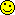<![CDATA[Math Is Fun Forum / collision]]> 2014-10-22T07:49:41Z FluxBB https://www.mathisfunforum.com/viewtopic.php?id=21542 <![CDATA[Re: collision]]> You are welcome.If you ever find out how this question was expected to be done, please let me know.

Bob

]]>
https://www.mathisfunforum.com/profile.php?id=67694 2014-10-22T07:49:41Z https://www.mathisfunforum.com/viewtopic.php?pid=338385#p338385
<![CDATA[Re: collision]]> thank you so much bob.]]>
https://www.mathisfunforum.com/profile.php?id=198409 2014-10-22T03:11:47Z https://www.mathisfunforum.com/viewtopic.php?pid=338376#p338376
<![CDATA[Re: collision]]> hi Niharika,

When I was taught this it was called Newton's experimental law.  If two balls collide with velocities u1 and u2 before the impact and v1 and v2 afterwards, then the coefficient of restitution is given by

and the law of conservation of momentum is

In real life 0 < e < 1.  But the kinetic theory of gases assumes that for molecules e = 1.

For the impact of the ball with the ground, e must be 1 for otherwise the ball will not climb back to its original height.  As we are not told the value of e for the impact with the block, I am assuming it, also, must be 1.  There's no way to do the problem without knowing e, because it affects the velocity that the ball bounces off the block.

By taking e = 1 we get

Substituting the given velocities (and taking right movement as positive):

So the ball bounces back with a horizontal component of velocity of 3v/2.

Alternatively, what happens if e = 0.5, say.

So the ball bounces back with a horizontal component of velocity of 7v/8.

So you can see the value of e is critical.

That's the best I can do with the information given in the problem.

Bob

]]>
https://www.mathisfunforum.com/profile.php?id=67694 2014-10-19T15:05:23Z https://www.mathisfunforum.com/viewtopic.php?pid=337954#p337954
<![CDATA[Re: collision]]> bob bundy wrote:

Now for the horizontal motion.  At impact with the block, the velocity is still V and the block is moving at -V/4.  We aren't told the mass of the block so no momentum equation is possible.  But we can use the elasticity equation.  We'll have to assume e=1  again as we aren't told anything else.  So

You'll need to be careful with negatives here, but you should be able to get the velocity of the ball after the impact (assume block is still -v/4).

Bob

I am not sure if I could understand the way of using that equation properly.

Will you pls show how you placed all the terms in that.

]]>
https://www.mathisfunforum.com/profile.php?id=198409 2014-10-19T10:42:27Z https://www.mathisfunforum.com/viewtopic.php?pid=337900#p337900
<![CDATA[Re: collision]]> hi Niharika,

Any information about 'coefficient of restitution' ?  ie.  How 'elastic' are the collisions  ?

I could assume it's the same for the block and the floor and hope it will cancel out I suppose.

More thinking:  If e = 1 for perfectly elastic collisions, then the ball would come back to its starting position without A moving at all.  As A is moving, this suggests that the energy lost during the collisions is exactly the same as the energy imparted to the ball by the block.  Hhhmmmm.LATER EDIT:

Ok.  I've thought about it.  Let's just think about the vertical motion first.

The initial velocity is zero and the ball accelerates under gravity.  When the ball hits the wall the vertical velocity is unaffected because it is parallel to the plain of the block and perpendicular to the motion of the block.  So we can just treat the vertical motion without worrying about the block at all.

So use

to get the time to the bottom.

Now the ball will only rise up to the start point if no energy is lost on impact with the ground, so the collision must be perfectly elastic (e=1).  This means the time for the ball to rise up is the same as the time to drop, so you can get the total time by doubling.

Now for the horizontal motion.  At impact with the block, the velocity is still V and the block is moving at -V/4.  We aren't told the mass of the block so no momentum equation is possible.  But we can use the elasticity equation.  We'll have to assume e=1  again as we aren't told anything else.  So

You'll need to be careful with negatives here, but you should be able to get the velocity of the ball after the impact (assume block is still -v/4).

So now you can get the total time for the two horizontal motions and hence get an equation for x in terms of everything else.

Bob

]]>
https://www.mathisfunforum.com/profile.php?id=67694 2014-10-18T16:18:32Z https://www.mathisfunforum.com/viewtopic.php?pid=337855#p337855
<![CDATA[collision]]> The block A begin to move with a velocity v/4.At the same time a ball is thrown from the wall of height h with a velocity v which traces the path  as shown before coming back to the original position.Find x.]]>
https://www.mathisfunforum.com/profile.php?id=198409 2014-10-18T15:10:40Z https://www.mathisfunforum.com/viewtopic.php?pid=337853#p337853